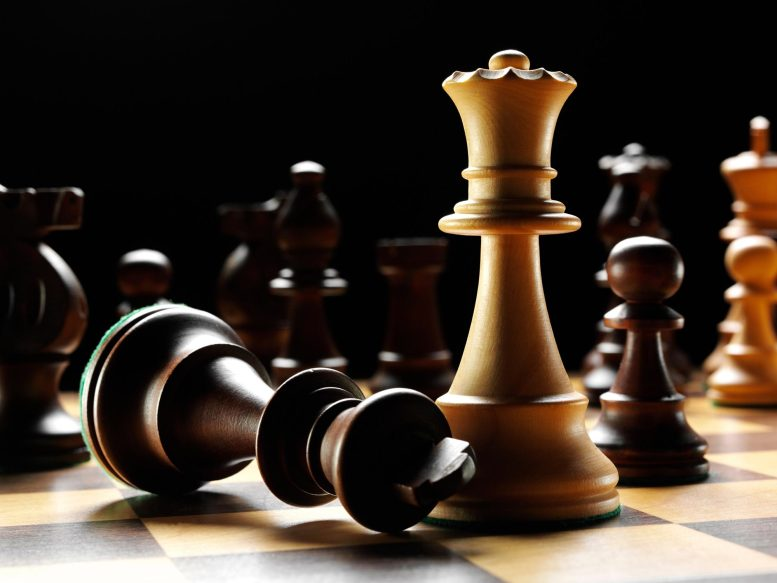# Harvard Mathematician Resolves 150-Year-Old Chess Problem,Harvard mathematician largely resolves 150-year-old chess problem involving most powerful piece on board.,The queen is the most powerful piece on the chessboard. Unlike any other (including the king), it can move any number of squares vertically, horizontally, or diagonally.,Now consider this queen’s gambit: If you put eight of them on a standard board of eight squares by eight squares, how many ways could they be arranged so that none could attack the other? Turns out there are 92. But what if you place an even larger number of queens on a chessboard of the same relative size, say, 1,000 queens on a 1,000-by-1,000 square chessboard, or even a million queens on a similarly sized board?,The original version of the n-queens mathematical problem first appeared in a German chess magazine in 1848 as the eight-queens problem, and the correct answer emerged a couple of years later. Then in 1869, the more expansive version of the problem surfaced and remained unanswered until late last year, when a Harvard mathematician provided an almost definitive answer.,Michael Simkin, a postdoctoral fellow at the Center of Mathematical Sciences and Applications, calculated that there are about (0.143n)n ways the queens can be placed so none are attacking each other on giant n-by-n chessboards.,Simkin’s final equation doesn’t provide the exact answer but instead simply says this figure is as close to the actual number as you can get right now. The 0.143 figure, which represents an average level of uncertainty in the variable’s possible outcome, is multiplied by whatever n is and then raised to the power of n to get the answer.,On the extremely large chessboard with one million queens, for example, 0.143 would be multiplied by one million, coming out to about 143,000. That figure would then be raised to the power of one million, meaning it’s multiplied by itself one million times. The final answer is a figure with five million digits.,Simkin was able to come up with the equation by understanding the underlying pattern for how large numbers of queens would have to be distributed on these enormous chessboards — whether they’d be concentrated in the middle or on the edges — and then applying well-known mathematical techniques and algorithms.,“If you were to tell me I want you to put your queens in such-and-such way on the board, then I would be able to analyze the algorithm and tell you how many solutions there are that match this constraint,” Simkin said. “In formal terms, it reduces the problem to an optimization problem.”,By focusing on the spaces that have the greater chances of being occupied, Simkin figured out how many queens would be in each section of the board and came up with a formula for to get a valid number of configurations. The calculations resulted in what’s known as the lower bound — the minimum number of possible configurations.,Once he had that number, Simkin then used a strategy known as the entropy method to find the upper bound, which is the highest number of possible configurations.,Simkin found the lower bound answer almost perfectly matches the upper bound answer. Simply put, it showed that the exact answer is sandwiched somewhere in between the two bounds in a relatively small mathematical space.,Simkin has been working on the
n-queens problem for almost five years. He says that he personally is a terrible chess player but is seeking to improve his game. “I still enjoy the challenge of playing, but, I guess, math is more forgiving,” said Simkin, who became interested in the problem because of how he could apply breakthroughs from the field of math he works in called combinatorics, which focuses on counting and problems of selection and arrangements.,Working on the problem has been a test of patience and resilience. Four years ago as a Ph.D. student at Hebrew University of Jerusalem, he visited mathematician and chess wiz Zur Luria at the Swiss Federal Institute of Technology in Zurich. The pair collaborated and developed new techniques to get at an answer. In the end, after two years of work, they only came up with
a better lower bound figure and knew they were missing something.,Simkin finished his Ph.D. in 2020 and moved to Boston to start working at Harvard. The problem was always at the back of his mind, and he came back to it when he realized he had to start focusing on spaces the queens would be rather than giving equal weight to each space.,Even though it’s theoretically possible to get a bit closer to an even more exact answer, Simkin for now is happy to let someone else come to it.,“I think that I may personally be done with the
n-queens problem for a while, not because there isn’t anything more to do with it but just because of I’ve been dreaming about chess and I’m ready to move on with my life,” he said.,Reference: “The number of n-queens configurations” by Michael Simkin, 19 August 2021,
Mathematics > Combinatorics.

arXiv:2107.13460,